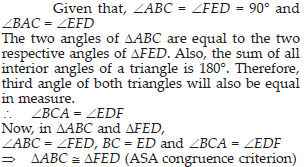Congruence of Triangles (Maths) Class 7 - NCERT Questions
Q 1.

Complete the following statements:
(A) Two line segments are congruent if ____________ .
(B) Among two congruent angles, one has a measure of 70°; the measure of the other angle is _______ .
(C) When we write ∠A = ∠B, we actually mean ________ .

SOLUTION:

(A) They have the same length.
(B) 70°
(C) m∠A = m∠B

Q 2.

Give any two real-life examples for congruent shapes

SOLUTION:

(i) Sheets of same letter pad.
(ii) Biscuits in the same packet.

Q 3.

If ∆ABC ≅ ∆FED under the correspondence ABC ↔ FED, write all the corresponding congruent parts of the triangles.

SOLUTION: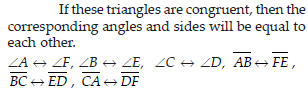Q 4.

If DDEF ≅ ∆BCA, write the part(s) of ∆BCA that correspond to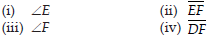SOLUTION: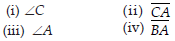Q 5.

Which congruence criterion do you use in the following?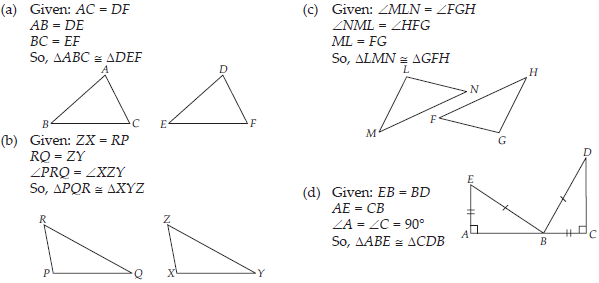SOLUTION:

(A) SSS, as all three sides of ∆ABC are equal to the corresponding sides of ∆DEF.
(B) SAS, as two sides and the angle included between these sides of ∆PQR are equal to the corresponding sides and included angle of ∆XYZ.
(C) ASA, as two angles and the side included between these angles of ∆LMN are equal to the corresponding angles and included side of ∆GFH.
(D) RHS, as in the given two right-angled triangles, one side and the hypotenuse are respectively equal.

Q 6.

You want to show that ∆ART ≅ ∆PEN,
(A) If you have to use SSS criterion, then you need to show
(i) AR =
(ii) RT =
(iii) AT =
(B) If it is given that ∠T = ∠N and you are to use SAS criterion, you need to have
(i) RT = and (ii) PN =
(C) If it given that AT = PN and you are to use ASA criterion, you need to have
(i) ? (ii) ?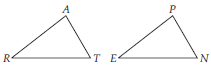SOLUTION:

(A) (i) AR = PE (ii) RT = EN (iii) AT = PN
(B) (i) RT = EN (ii) PN = AT
(C) (i) ∠ATR = ∠PNE (ii) ∠RAT = ∠EPN

Q 7.

You have to show that ∆AMP ≅ ∆AMQ
In the following proof, supply the missing reasons.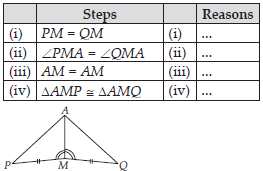SOLUTION:

(i) Given
(ii) Given
(iii) Common
(iv) SAS, as the two sides and the angle included between these sides of ∆AMP are equal to corresponding sides and angle of ∆AMQ.

Q 8.

In ∆ABC, ∠A = 30°, ∠B = 40° and ∠C =110°
In ∆PQR, ∠P = 30°, ∠Q = 40° and ∠R = 110°
A student says that ∆ABC ≅ ∆PQR by AAA congruence criterion. Is he justified? Why or why not?

SOLUTION:

No. This property represents that these triangles have their respective angles of equal measure.
However, this gives no information about their sides. The sides of these triangles have a ratio somewhat different from 1:1. Therefore, AAA property does not prove that the two triangles are congruent.

Q 9.

In the figure, the two triangles are congruent.
The corresponding parts are marked. We can write ∆RAT ≅ ?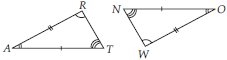SOLUTION:

It can be observed that,
∠RAT = ∠WON
∠ART = ∠OWN
AR = OW
Therefore, ∆RAT ≅ ∆WON, by ASA criterion.

Q 10.

Complete the congruence statement: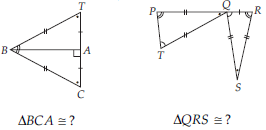SOLUTION: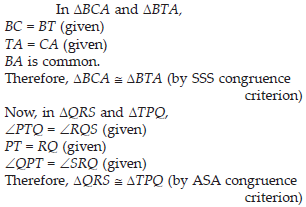Q 11.

In a squared sheet, draw two triangles of equal areas such that
(i) the triangles are congruent.
(ii) the triangles are not congruent.
What can you say about their perimeters?

SOLUTION: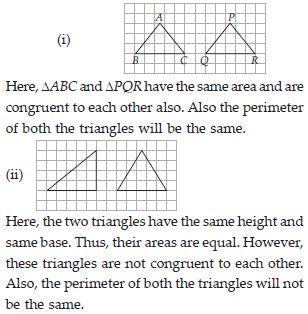Q 12.

Draw a rough sketch of two triangles such that they have five pairs of congruent parts but still the triangles are not congruent.

SOLUTION: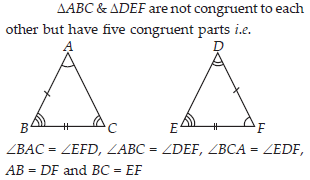Q 13.

If ∆ABC and ∆PQR are to be congruent, name one additional pair of corresponding parts. What criterion did you use?

SOLUTION: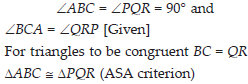Q 14.

Explain, why ∆ABC ≅ ∆FED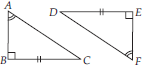SOLUTION: